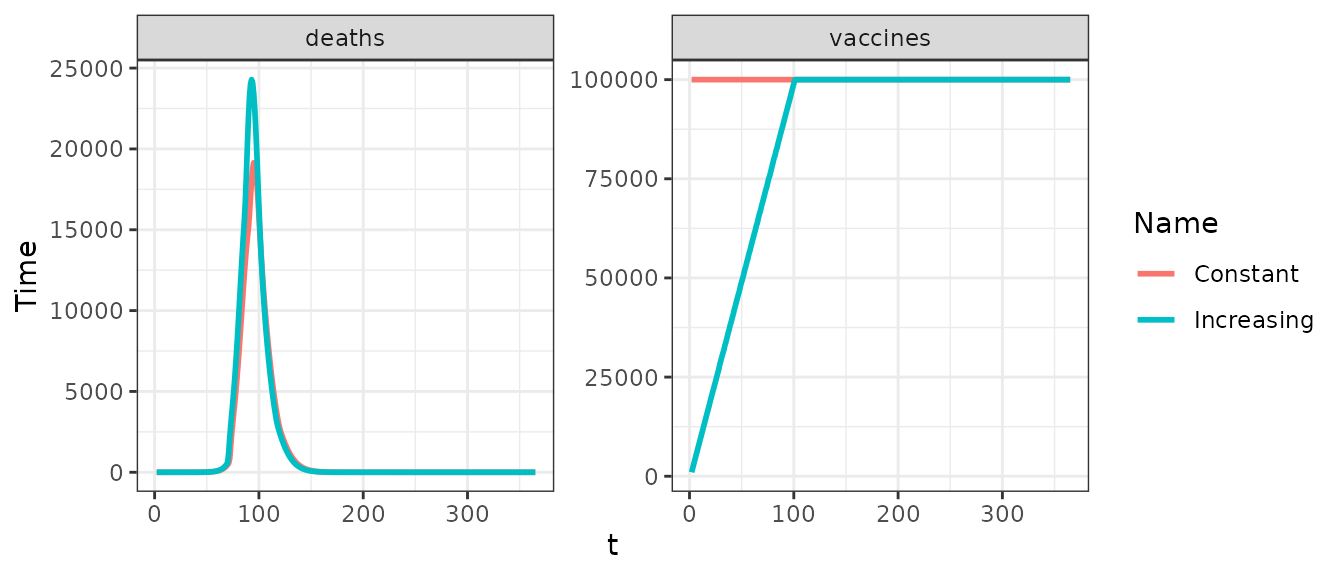library(nimue)
library(ggplot2)
library(dplyr)

We can model a scale-up of supply capacity for vaccines

### No initial supply constraint

To begin, we can run the model with no supply constraints - in this case we immediately have the ability to vaccinate 100,000 individuals per day:

# Run the with no vaccine supply constraint
constant <- run(
country = "United Kingdom",
max_vaccine = 100000,
vaccine_efficacy_disease = rep(0, 17),
vaccine_efficacy_infection = rep(0.9, 17)
)
# Format the output selecting vaccines and deaths
o1 <- format(constant, compartments = NULL, summaries = c("deaths", "vaccines")) %>%
mutate(Name = "Constant")

### Initial supply constraint

A more realistic scenario might see us ramp up our supply capcity over time, in this case ramping up to our target of vaccinating 100,000 people per day over a period of 100 days:

# Run with initial vaccine supply constraints
increasing <- run(
country = "United Kingdom",
max_vaccine = seq(0, 100000, length.out = 100),
tt_vaccine = seq(0, 100, length.out = 100),
vaccine_efficacy_disease = rep(0, 17),
vaccine_efficacy_infection = rep(0.9, 17)
)
# Format the output selecting vaccines and deaths
o2 <- format(increasing, compartments = NULL, summaries = c("deaths", "vaccines")) %>%
mutate(Name = "Increasing")

### Compare

# Create plot data.frame
pd <- bind_rows(o1, o2)
# Plot outputs
ggplot(pd, aes(x = t, y = value, col = Name)) +
geom_line(size = 1) +
facet_wrap(~ compartment, scales = "free_y") +
ylab("Time") +
theme_bw()